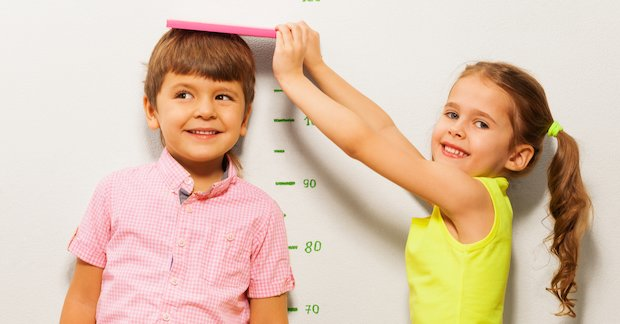# Mastery in Mathematics – Measurement Recommended

This content has not been rated yet. (Write a review)

Measurement is one of the most easily recognisable ‘real life’ aspects of mathematics and applying it to real life settings should be a key focus when teaching it. It doesn’t matter whether the focus is money, time, length, weight, volume or area, all these aspects of mathematics will have everyday applications throughout a person’s life.There are many aspects of measurement to master and it is perfectly conceivable that a child may find one aspect easier than another. In the modern age where time is seen in a digital format, for example, it is often difficult to overcome the abstract nature of analogue time with its multiple layers of complexity. Other more linear aspects like the order of length, eg 1000 mm = 100 cm = 1m can be more easily understood. This linearity exists with money, area, capacity and volume so it is important to link the use of measure as much as possible to the application of number being built upon. The use of examples using measure during the development of number is useful in making the links necessary for fluency in both areas later on.

### Progression in measurement

Measure can be split into different topics, length (and height), weight (and mass), capacity, volume and area. It is absolutely vital to link children’s knowledge in place value and their calculation skills when teaching these concepts. Place value underpins a developing understanding in conversion with measure as well as comparing, ordering, measuring and calculating different measurements, especially with perimeter, area and volume. It is essential to develop the use of the contrete, pictorial, abstract approach throughout the teaching of measurement, as this will help children to make the connections between different areas, provide hands on experience and develop their reasoning skills. Children need to:

• Use non-standard units of measure before moving on to comparing and describing and then ordering.
• Develop an understanding of standard units of measure.
• Develop an understanding of estimating different measure, measuring and recording.
• Be able to develop a greater understanding of place value, for instance being able to seeing that 1 mm < 1 cm and 1 ml < 1 l. This is especially important when converting between different metric measures, eg from cm to m and l to ml.
• Convert from metric measure to imperial measure and see the link between these.
• Understand length by:
• Using centimetres (cm) and metres (m) before moving onto looking millimetres (mm) and kilometres (km).
• Being able to measure and compare lengths using addition and subtraction.
• Developing an understanding of finding perimeter by measuring and adding the length of sides together, eg adding 3 sides together for a triangle.
• Finding the perimeter of rectilinear shapes before composite rectilinear shapes.
• Developing an understanding of area, firstly by counting the squares inside rectilinear shapes and then moving on to composite shapes and irregular shapes.
• Understanding that using the square symbol with different measure is how area is measured and recorded.
• Using formulae to find area and perimeter with the understanding that calculation is key to this.
• Using their understanding of area to find the area of triangles, parallelograms and other shapes.
• Understand weight (mass) by:
• Having hands on experience, eg balancing scales to develop concepts of heavy and light and equality.
• Using scaled measurements to further aid development in measuring and recording.
• Understand capacity and volume by:
• Using measuring equipment, such as measuring jugs, to understand concept of full, empty, half full, quarter full. This concept can also be linked to fractions.
• Developing an understanding of volume using cubed meausrement. Building models of cubes and cuboids will further develop an understanding of volume before looking at the volume of irregular shapes and using formulae.
• Understand time by:
• Developing an understanding of using hours, minutes and seconds.
• Telling the time to the hour, half past, quarter past and to smaller units of time using analogue then digital clocks.
• Developing an understanding of chronology such as days of the week, months and years and knowing how many hours/minutes/seconds are in a day etc.
• Developing an understanding of using Roman numerals for clocks and telling the time as well as understanding am and pm for the 12 hour clock and developing an understanding of the 24 hour clock.
• Converting between different units of time.
• Understand money by:
• Developing an understanding of pence and then pounds.
• Combining amounts to make values and using calculation skills to develop this.
• Problem solving using money and converting between amounts.
• Understand temperature by:
• Using equipment such as thermometers.
• Recording this using °c (degrees centigrade).
Member-only content

## Scholastic Resource Bank: Primary - join today!

• Over 6,000 primary activities, lesson ideas and resources
• Perfect for anyone working with children from 5 to 11 years old
• Unlimited access from just £1.25 per month